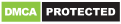### Write Comment

Type in
(Press Ctrl+g to toggle between English and the chosen language)

•Let ages of P,Q and R be $p,q,r$ respectively According to ques, => $(p+r)+2q=59$ ------------(i) and $(q+r)+3p=68$ -------------(ii) and $p+3q+3r=108$ ------------(iii) Multiplying equation (ii) by 3, => $3q+3r+9p = 204$ ----------(iv) Subtracting equation (iii) from (iv), we get : => $(9p-p)=(204-108)$ => $8p=96$ => $p=frac{96}{8}=12$ years => Ans - (D)Powered By:Omega Web Solutions